### LHComedy: CERN After Dark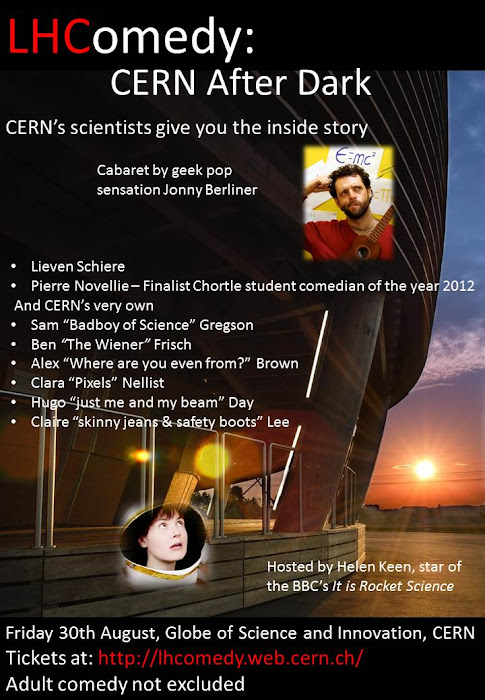LHComedy brings science-themed stand-up comedy to the Geneva area. Bringing our audience a mixture of laughs and insight, we bring our passion about science and technology to the world with our own stories and experiences of life at CERN.
(more at lhcomedy.web.cern.ch)

### Crazy space### Neutrinos: between Pontecorvo and Majorana

Neutrinos are the most elusive elementary particles in the whole zoo. The reasons are simple: first of all neutrinos don't have electric charge, so physicists cannot use electromagnetic experiments in order to detect them, and they must design indirect measures; furthermore they interact with other particles only with weak interaction. At the other hand, neutrino is, in Standard Model, massless, while from an experimental point of view, he has a really small mass: at the beginning of 2000, Mainz and Troitsk experiment measured a maximum value at 2.2 eV, that is about 4 milion less that the electron mass! The idea of neutrino's mass is dued by Bruno Pontecorvo that introduced in 1957 the so called neutrino's oscillations(1, 2): in this model is expected the existence of three type of neutrinos that, combining with each other, giving rise to neutrinos usually observed in experiments. The thoery was further developed in 1962 by Ziro Maki, Masami Nakagawa and Shoici Sakata(3): $\begin{pmatrix} \nu_e \\ \nu_\mu \\ \nu_\tau \end{pmatrix} = \begin{pmatrix} U_{e_1} & U_{e_2} & U_{e_3} \\ U_{\mu_1} & U_{\mu_2} & U_{\mu_3} \\ U_{\tau_1} & U_{\tau_2} & U_{\tau_3} \end{pmatrix} \begin{pmatrix} \nu_1 \\ \nu_2 \\ \nu_3 \end{pmatrix}$ where $e$, $\mu$, $\tau$ indicate the three different leptons (electron, muon and tau), $\nu$ are neutrinos, with $\nu_i$, where $i = 1,2,3$, the fundamental neutrinos.
But, if the Pontecorvo–Maki–Nakagawa–Sakata matrix describes neutrinos' oscillations, we could describe the neutrino also using a particular equation: the Majorana equation(4): $i \gamma^\mu \partial_\mu \psi - m \psi_c = 0$ where $\psi_c = \gamma^2 \psi^*$ is the so called conjugated charge.
Now, if a wave function $\psi$ respects the Majorana equation, then $m$ is called Majorana mass; if $\psi$ coincides with $\psi_c$, then $\psi$ is said Majorana spinor; finally, if there is a particle that can be described with the Majorana equation, then this is called a Majorana particle, i.e. a particle that coincides with its antiparticle. The leading candidate to be a Majorana particle is, look at the case, the neutrino, whose mass is probably not so important with regard to the ultimate fate of the universe. In fact, the astronomical data suggest a flat universe, where flat universe means a substantial balance between gravitational attraction and expansion of spacetime.
Conclusion: the importance of neutrino oscillations are related to the property to possess a mass: experiments confirmed that property, owned by all three neutrinos in the game. The astronomical data, however, assign this property a minor role for the ultimate fate of the universe, while its mass shows instead of the Standard Model, at present, still does not understand much of the physics of our universe. Among the facts not included in the Standard Model are the Majorana particles: in particular, the neutrino could be one of them and if this is confirmed, then we would have a great step in order to know the symmetry breaking between matter and antimatter.

### What makes a good math teacher?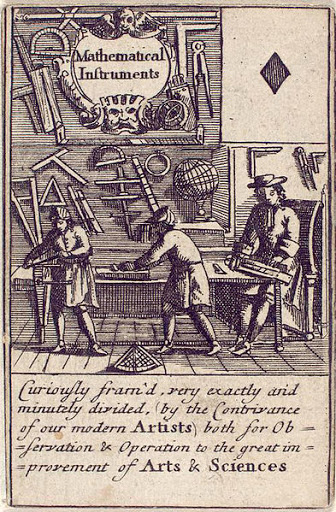• Motivates students to learn by making the subject interesting;
• Is knowledgeable;
• Presents the material clearly;
• Is available to students;
• Is intellectually challenging;
• Cultivates thinking skills;
• Sets high standards; and
• Encourages self-initiated learning.

### L1448-MM: water in space

One of the most interesting research topics is the star formation. The high weigth chemistry elements could diffusein the universe only with the life cycle of the stars: the birth, the syntesis of helium and of the other elements, the great explosion that (diffusero) in space carbon, iron and so on. But also the early stages in star formation could send in cosmic vacuum some light elements like hydrogen, or also water!
One place in which it can observe not only star formation but also water jets is the L1448 dark cloud in Perseus molecular cloud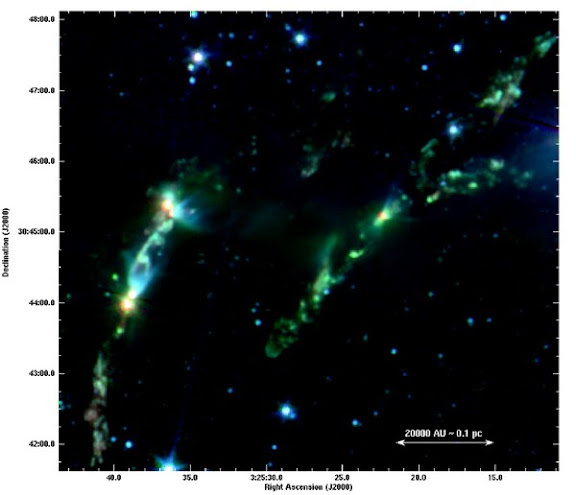In particular there is an interesting object, L1448-MM that presents two bright spots of emission, the cross (A) and the star (B) in the following figure

### Carl Sagan explains Flatland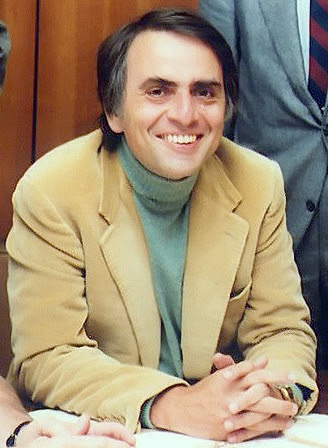### The B mesons and the new physics

posted by @ulaulaman via @LHCbExperiment #newphysics #Bmesons #LHC #CERN #particlephysics
The search about B mesons decays has a great importance in physics for the possible clues of new physics that could be discovered. So theoretical phisicists have developed some new observables in order to test this possibility. LHCb produced new results about these new parameters, in particular the so called $P_5'$.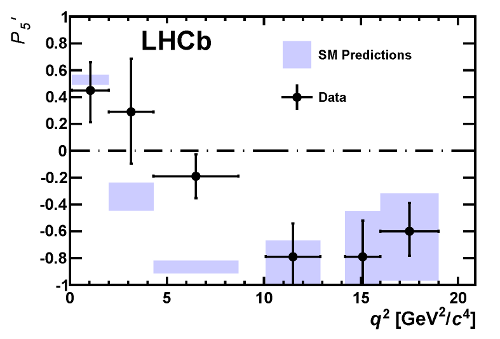According to Joaquim Matias from Universitat Autonoma de Barcelona and colleagues the deviation in $P_5'$ and small discrepancies in the other angular observables for this decay, follow a pattern. In a recent paper the authors claim that a global analysis of the LHCb data, together with previous measurements, show a deviation of $4.5 \sigma$ with respect to Standard Model expectations, which can be explained with the same mechanism. This demands further investigation, in particular to re-evaluate all the sources of theoretical uncertainty, and to understand the effects of correlations between the experimental measurements.
(via LHCb)
The image shows the distribution of the $P_5'$ observable as a function of the $\mu^+ \mu^-$ invariant mass squared $q^2$. The black data points are compared with the Standard Model prediction.

### Paul Dirac and the relativistic world

posted by @ulaulaman #PaulDirac #DiracDay #DiracEquation #Klein-GordonEquation #relativity #physics
It seems to be one of the fundamental features of nature that fundamental physical laws are described in terms of a mathematical theory of great beauty and power, needing quite a high standard of mathematics for one to understand it.
(Paul Dirac)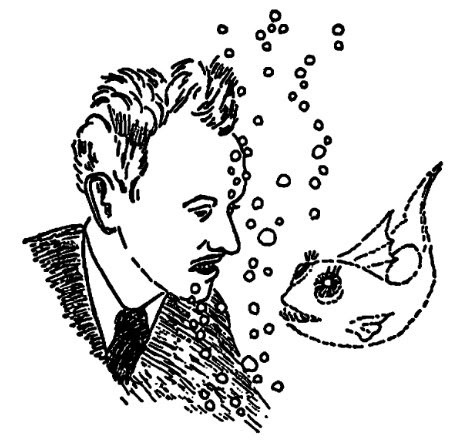For a physicists the beauty of an equation is often in its conciseness and in the amount of physical information that is able to synthesize, and so the wide variety of systems that it can be represented. For example, the Schrodinger equation, in its most synthetic form, is able to describe a disparate number of systems, and only descending the details of each system, physicists are able to describe the system with with more or less complicated solutions. $i \frac{\partial}{\partial t} \psi = H \psi$ Something like that it's maked by the equations discovered by Klein-Gordon and Dirac, but for the relativistic motion: indeed particles are able to travel even at near the speed of light, and in that case the Schrodinger equation is no longer sufficient to describe their dynamics.
From this we need to describe the world from the point of view of quantum relativistic: the Klein-Gordon equation is born: $\partial^\mu \partial_\mu \phi + m^2 \phi = 0$ It has two drawbacks: negative energy as a solution and interpretation of the wave function. If, as in quantum mechanics, we associate the wave function with probability, or rather the probability's density, it happens that this can also take on negative values. At this point arrived the Dirac equation: $\left ( i \gamma^\mu \partial_\mu - m \right ) \psi = 0$ Thanks to the Dirac equation, whose one of the first success was the explanation of half-integer spin of the electron, we now have two fundamental tools: the first for the description of bosons (particles with integer spin), the second for the description of fermions (half-integer spin particles).
Read also: The Relation between Mathematics and Physics, a lecture by Dirac
In the image: Paul Dirac by George Gamow

### Turing, Fibonacci and the sunflowers

posted by @ulaulaman about #FibonacciDay #AlanTuring #TuringSunflowerToday is the american Fibonacci's day, so it could be a good day to write something about one of the last work by Alan Turing.
One of a number of problems [Alan Turing] was trying to solve was the appearence of Fibonacci numbers in the structure of plants.(1)
The problem was knwon as the Fibonacci phyllotaxis, and we can state it in this way:
the spiral shapes on the heads of sunflowers seemed to follow the Fibonacci sequence, prompting [Turing's] proposal that by studying sunflowers we might better understand how plants grow
Turing wrote his interest in a letter to the zoologist JZ Young: About the point (iii) Turing wrote in another letter:
Our new machine is to start arriving on Monday. I am hoping to do something about 'chemical embyology'. In particular I think I can account for the appearence of Fibonacci numbers in connection with fir-cones.(1)
The last year Jonathan Swinton, during the Manchester Science Festival in October, announced the results of the great experiment about the Turing's sunflower: